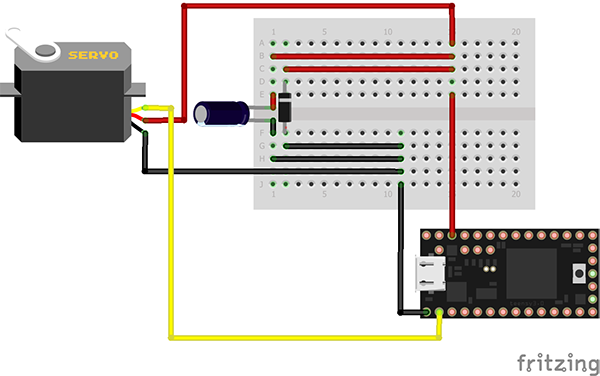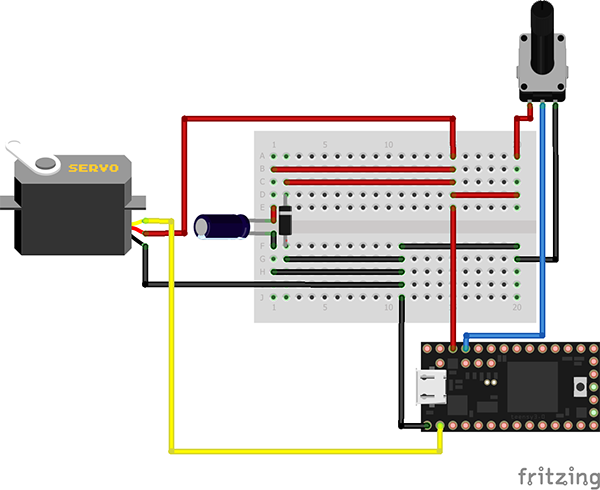Heya, Thanks for visiting!

# CNC: Controlling Linear Servos

• teensy-3
• cnc
• servo

Here is a simple guide/tutorial on how to get your linear servo moving. The video below demos what I made. You can see how everything is set up below that:

Play video

# Parts:

• Teensy 3.0 or 3.1: Substitute your own Arduino/microcontroller
• Mini Linear Servo
• 1x 10 uF Capacitor
• 1x Diode
• (Optional) Potentiometer for jog code.

I was able to run the mini servo off of the Teensy's 3.3V pin which has a 100mA max rating.

# Code:

There are two different demo projects. The first one just jogs the servo back and forth. The second demo uses the potentiometer as input to manually control the position of the servo.

A linear servo works just the same as a normal hobby 0 to 180 degree servo. Instead of a short pulse being 0 degrees it represents a bottom position and 180 degrees represents a top position.

Pulse relationship (linear):

• 1000 microseconds: bottom position
• 1500 microseconds: middle position
• 2000 microseconds: top position

The diode in both designs acts as a flyback diode to mitigate any current generated from moving the servo by hand (bumping the arm, etc).

## First demo:

Sweeps the linear servo up and down.``````int servoPin = 0;
int pulse = 1000;

void setup() {
pinMode(servoPin, OUTPUT);
}

void loop() {

digitalWrite(servoPin, HIGH);
delayMicroseconds(pulse);
digitalWrite(servoPin, LOW);

delay(300);

digitalWrite(servoPin, HIGH);
delayMicroseconds(2000);
digitalWrite(servoPin, LOW);

delay(300);

}``````

## Second Demo:

Manually control/jog the linear servo with the potentiometer. Includes some averaging to smooth the potentiometer input.``````const int servoPin = 0;
const int potPin = 23;

const int pulseStart = 1000; // Number of microseconds to get to position 0%
const int pulseEnd = 2000; // Number of microseconds to get to position 100%
int pulseRange = -1; // We use this later to scale potval

const int timeBetweenPulses = 30000; // Number of microseconds between pulses

elapsedMicros sincePulse; // Keeps track of time between pulses
elapsedMicros sincePulseStart; // Keeps track of pulse length in time
elapsedMicros sinceSerial;

int potVal = 0;

int total = 0;

int averagedPotVal = 0;
int pulseTime = 0;

void setup() {
pinMode(servoPin, OUTPUT);

pulseRange = pulseEnd - pulseStart;

for(int i = 0; i < numReadings; i++)

Serial.begin(9600);
}

void loop() {

{

// advance to the next position in the array:

// if we're at the end of the array...
// ...wrap around to the beginning:

// calculate the average:

pulseTime = (((float)averagedPotVal/1023)*pulseRange)+pulseStart;

}

if (sinceSerial >= 100000)
{
String unformater = "'";
String sep = ", ";
Serial.println(String(averagedPotVal) + sep + String(pulseTime) + "\0");
sinceSerial = 0;
}

if(sincePulse >= timeBetweenPulses)
{
// Keep high for the amount we want
if (sincePulseStart <= pulseTime)
{
digitalWriteFast(servoPin, HIGH);
}
else
{
digitalWriteFast(servoPin, LOW);

// No need to to do this as we do it later
// But doesn't hurt
sincePulseStart = 0;

// Now that the pulse is done
// Reset the time between pulse
sincePulse = 0;
}

}
else
{
// Reset pulseStart time until we actually get to doing it
// Because this variable keeps counting
sincePulseStart = 0;
}

}``````